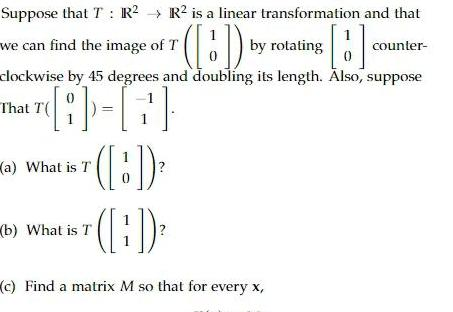Question:

# R is a linear transformation and that BD by rotating B

Last updated: 9/10/2023R is a linear transformation and that BD by rotating B counter clockwise by 45 degrees and doubling its length Also suppose That T T Suppose that T R we can find the image of T a What is T b What is T 1 1 c Find a matrix M so that for every x